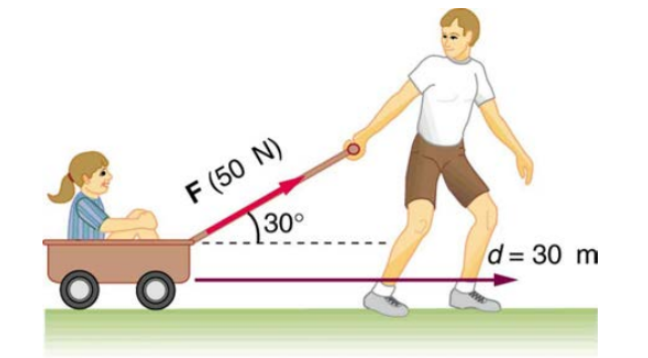# Problem: How much work is done by the boy pulling his sister d = 30.0 m in a wagon as shown in figure below? Assume no friction acts on the wagon and he pulls with a force of 50.0 N and at an angle θ = 30°.

###### FREE Expert Solution

Work:

$\overline{){\mathbf{W}}{\mathbf{=}}{\mathbf{F}}{\mathbf{d}}}$

Force applied by the boy is the horizontal component of the force.

FH = F cos θ

96% (364 ratings)###### Problem Details

How much work is done by the boy pulling his sister d = 30.0 m in a wagon as shown in figure below? Assume no friction acts on the wagon and he pulls with a force of 50.0 N and at an angle θ = 30°.